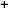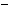Labware - MA35 Multivariable Calculus - Polar Coordinate Labs
 MA35 Labs » Polar Coordinate Labs Contents.4 Integration .4.1 Volume Under a Function Graph .4.2 Riemann Integral Search

Volume Under a Function Graph

Text

Just as in the Cartesian case, the definite integral of a function of two variables in polar coordinates represents the volume underneath its three-dimensional function graph.Recall that the procedure for evaluating single variable integrals is to
1. approximate the area under the graph using rectangles; and
2. take the limit of the sum of the area of these rectangles as the number of rectangles approaches infinity.

Similarly, the volume underneath the function graph for a double integral can be found by
1. dividing the domain R into rectangles;
2. erecting rectangular prisms over these rectangles using the value of the function graph at the bottom-left vertex of each rectangle as the height;
3. taking the limit of the sum of the prisms volumes as the number of rectangles in the domain aproaches infinity.Demos

 Approximation by Rectangular Prisms